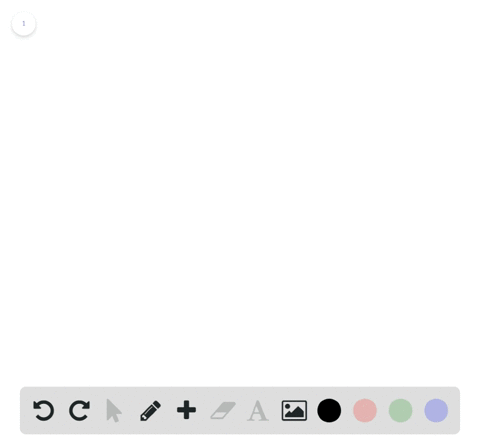Enroll in one of our FREE online STEM bootcamps. Join today and start acing your classes!View Bootcamps01:24
Problem 52

# Using the $\varepsilon$ -\delta Definition of Limit In Exercises $45-56$ , find the limit $L$ . Then use the $\varepsilon-\delta$ definition to prove that the limit is $L$ .$$\lim _{x \rightarrow 4} \sqrt{x}$$

## Discussion

You must be signed in to discuss.

## Video Transcript

in this problem, we want to show that the limit is X approaches for of the square root of X is equal to two. We want to do this using that slammed out to definition of a limit. So that means we want to show effort. Every Absalom greater than zero there is adult greater than zero such that thie, absolute value of the square root of X minus two is less than epsilon. Whenever the square root of X minus four is in between zero and delta time. So you know. So this here is the inequality that we want to satisfy and we want to find tell to that would always go always work the matter which positive number Absalon ticks on. So this here is true then, yeah, we can take both sides of this equation and multiply them by the conjugation of thie absolute value of the square root of X minus two. So we kind of multiply besides by the conscript of it's great of X minus two and noticed that the Contra kit is in absolute value. So it's always positive, so we don't have to worry about flipping this inequality sign or anything like that. So since that's true and we combine, these terms will see that they actually equal. He have some value of X minus four. Yeah, So that tells us that that is less than Absalon Times, the Contra kit. So now we have this expression here in terms of absolute and X two variables and three what we wanted. Teo is bound one of them, so we're going to bound X so that it's somewhat fixed. So let's let X be in the interval one tonight so that it must be the case that the square root of X is in the interval. 123 and the square root of X close to is in the interval 3 to 5. Because square root of X is just shifted over on the number line by two silly kind of add to to the end points. And here we kind of take discovered of the end points. So an interval notation. This says that three is less than this query to vex close to when that's less than five. There's also implies by taking reciprocal is that 1/3 is greater than one over the square root of X plus two when that's creator and one over five. So this will be very important to us in a few steps. So no, let's let UPS alone be given. And let's let Delta equal to three times up Salon. Then, if we have that, zero is less than that's the value of X minus four, which is always true since the absolutely anything should be greater than zero. And that's less than Delta, which is three times up. Salon. It must be the case that the factory ization of X minus four, which we saw right here, is also in that interval because they're equal, sir. So we've kind of just written this absolute value in its factored form. So this implies that this value right here it's less than three absalon divided by this conjugal value here, if we kind of divided over on both sides, therefore we have that absolute value of the square root of X minus two is less than three Absalon divided by this value. Remember, we have at this is bounded by 1/3 has bounded above by 1/3. So that's less than three Absalon times 1/3 which, of course, is less than absolute when we're done. So you picked experience for once. Nine. If Absalon, we've chosen to be particularly large, we might run into some issues, but we can always adjust and pick a small enough delta to match the up salon because of this relationship here.